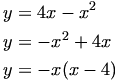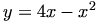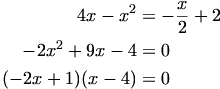## Maths Help Line Qs

### Rocky

Posted 15 September 2005 - 03:30 PM

1- The parabola shown has equation "y= 4x - x2 and intersects the x-axis at the Origin and the Point P.
It has a Max T.P. as -x2 and on right part of parabola when falling down and cuts x-axis, is point P. In paraobla on top left has point Q. And if you follow line it cuts y-axis which is R.
(a) Find the Co-ords of P
(b) R is the point (0, 2)
Find the equation of PR
© The line and the parabola intersect at Q.
Find the Co-ords of Q

2- The 2 lines AB and ED cross at the point T.
A is (0, 2), B(11, 7), E(4, 1) and D(7, 8).
(a) Wrtie down the gradients of the lines AB and ED.
(b) Hence find the size of the acute angle DTB between the 2 lines.

### Rocky

Posted 15 September 2005 - 05:03 PM### dondon

Posted 15 September 2005 - 06:14 PM

I have absoloutely no idea about the first question mostly because you wrote y=4x-x2
what did you mean by x2??

But
2) for the firstr part you just use the equation y-y/ x-xand put in the points so it works outt as

m(AB) =5/11
m(DE) =7/3
but you will need to show working
do you know how to do this because youveasked a few times about how to work out gradients?!

b)
I thought i could remember how to do this but i cant all i know is its got something to do with tan in itsomeone should know though!

### dfx

Posted 15 September 2005 - 06:15 PM

I'll give you a start:

I'll presume what you meant was:So it crosses the x-axis at 4 and the origin. From what you've described, the point P would be (4,0).

(b) Should now be VERY straightforward.

( c ) Intersection. Whenever that word appears, you equate the two equations and set = 0 and find values of x. There's alot of intersection questions in the Higher.

2.

(a) Oh come on!

(b) That looks like a vectors question. Doubt you'll be able to do that right now, unless you've done unit 3.

### Rocky

Posted 21 September 2005 - 04:31 PM

I got -2(x2) -7x + 2=0

How do you factorise this as I can't get it to work.

### dfx

Posted 21 September 2005 - 05:07 PM

Are you sure your line equation is correct?

I've got P = (4,0), R = (0,2). Then the gradient is -1/2. And the line equation turns out as y = (-1/2)x + 2.

Equating this withx = 1/2, x = 4.

From this, it should be fairly obvious by looking at the diagram which of the 2 points Q actually is. If I've figured your diagram out correctly, it should be x = 1/2, since you say Q is at the "top left", and the curve cuts the x-axis at x = 4. Whatever the value, plug it into the line PR or curve equation - whichever you prefer - and get the corresponding y value. And you get your Q.

### Rocky

Posted 22 September 2005 - 03:13 PM

So I can sub 4 into the equation of line PR ( y=-1/2x + 2 ). And it won't matter if I don't put 1/2 instead into it? As you get different answers, as want to know. Would it matter?

*Also Q-2. (a) I got gradients AB=5/9 and ED=7/3.

In part (b) it says 'Hence use this to find the acute angle between the 2 lines. So how you do that, I thought maybe 'exact values' Q, but it isn't as no square roots. So any idea? As I don't think it's a vector Q.

### dondon

Posted 22 September 2005 - 03:56 PM

For the first part youve copied the equation wrong itsy = (-1/2)x + 2., which although it doesnt look that different it can make a big difference.

No it doesnt matter that you get different answers these are the y coordinates of the number you put in.

Im not very sure about the second part but i'm sure you do something with tan, the problem is i threw out all my maths stuff so im not really completely sure how to do it.
But i'm sure someone else will be able to.

### Rocky

Posted 22 September 2005 - 04:15 PM

Ok, just as Q 2 is a kind of exact values Q. But it isn't though.

### Dave

Posted 22 September 2005 - 04:21 PM

might i suggest you look up your notes for that question i am sure its a learning outcome to be able to do that question

actually yes are you sure you have to do that question because i remember in higher there was questions in the book that we couldnt do because they were work from other units

### Rocky

Posted 22 September 2005 - 04:40 PM

From your experience, do you know how to do this Q? As my notes have this Q but its with 'Exact Values' dude. And this Q ain't though### dfx

Posted 22 September 2005 - 05:16 PM

Look up in your coordinate geometry in the different methods to calculate gradients section. Hint: relationship between tan and gradients. And thereforth it's just applying simple logic. If you get stuck, post back.

### Rocky

Posted 23 September 2005 - 02:36 PM

I'm stumped so enlighten me dude. lol

### Rocky

Posted 23 September 2005 - 03:55 PM

As I've looked at my notes and tried it so can anyone help please.

### Dave

Posted 23 September 2005 - 04:56 PM

m = tanfor the angle between a line and the x axis

once you have that angle then use your rules of triangles and co-ordinating angles to find DTB

### dfx

Posted 23 September 2005 - 05:11 PMThe gradient of any line is also the tangent of the angle that line makes with the x-axis. So take the tan inverse of the gradient, and you get the angle it forms with the x-axis. I've indicated the angles above, along with the intersection. The angle you're looking for is DTB - not clearly indicated, but I think you really should know that. You should now be able to figure it out. Also, when solving straight line/graph/intersection questions, often a very quick sketch of the graph is extremely helpful in visualizing what's going on, so try getting used to that.

A really useful tool if you're going to be doing Higher/Advanced Higher math is zap-a-graph which lets you do alot of sketching...

http://home.istar.ca/~bwaves/ZAPGRAPH.ZIP

Nonetheless, really do try to learn how to make quick sketches - you'll need it. Post back if there's any probs.### dfx

Posted 23 September 2005 - 05:19 PM

ps: the angle which you get from the tan inverse of the gradient, is the angle that the line makes with the POSITIVE side of the x-axis. Just incase you were wondering why it wasn't the other side.

### Rocky

Posted 24 September 2005 - 12:31 PM

Hey, couldn't download that thing as went on wordpad, then wierd writing came on it. So listen how did you get 66.8 and 24.4? As like to how you worked it out, then afterwards how do i use it to find DTB.

### dfx

Posted 24 September 2005 - 01:16 PM

Read the above posts carefully.

Basically:

The GRADIENT of any line, is the TANGENT of the ANGLE that line makes with the positive direction of the x-axis. So if the gradient is the tangent of the angle, and you want the angle, you simply take the inverse tan of the gradient, comprendo?

So in the above case we just take the< that's inverse Tan, of the gradients for AB and DE (using a calculator, ofcourse.) We then know the values of the angles which turn up as 66.8 and 24.4 degrees.

As for the angle DTB:

Keep in mind that the sum of the angles in a triangle is 180 degrees. Also remember that the angle ae straight line is 180 degrees. The x-axis is your straight line. Now you should be able to figure it out.